# Adjacent Angles & Vertical Angles

The measure of rotation of a ray, when it is rotated about its endpoint is known as the angle formed by the ray between its initial and final position. At times, in geometry, the pair of angles are used. There are various kinds of pair of angles, like – supplementary angles, complementary angles, adjacent angles, linear pair of angles, opposite angles, etc. In this article, we are going to discuss the definition of adjacent angles and vertical angles in detail.

The two angles are said to be adjacent angles when they share the common vertex and side. The endpoints of the ray form the side of an angle is called the vertex of a angle. Adjacent angles can be a complementary angle or supplementary angle when they share the common vertex and side.

Consider a wall clock, The minute hand and second hand of clock form one angle represented as ∠AOC and the hour hand forms another angle with the second hand represented as∠COB. Both these pair of angles i.e.∠AOC and ∠COB lie next to each other and are known as adjacent angles.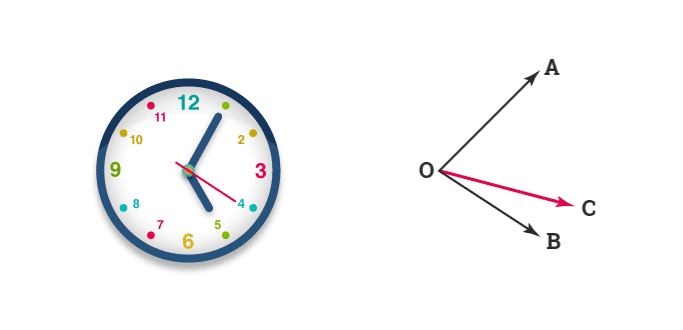∠AOC and ∠COB have a common vertex, a common arm and the uncommon arms lie on either side of the common arms. Such angles are known as adjacent angles.

Some of the important properties of the adjacent angles are as follows:

Two angles are adjacent-angles, such that

• They share the common vertex
• They share the common arm
• Angles do not overlap
• It does not have a common interior-point
• It can be complementary or supplementary angles when they share the common vertex.
• There should be a non-common arm on both the sides of the common arm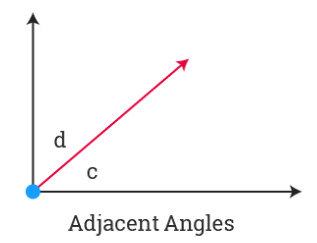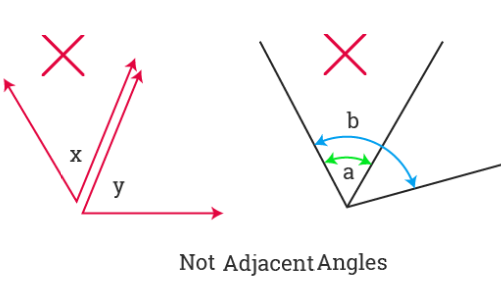What is the sum of adjacent angles? The adjacent angles will have the common side and the common vertex. Two angles are said to be supplementary angles if the sum of both the angles is 180 degrees. If the two supplementary angles are adjacent to each other then they are called linear pair.

Sum of two adjacent supplementary angles = 180o.

Here are some examples of Adjacent angles: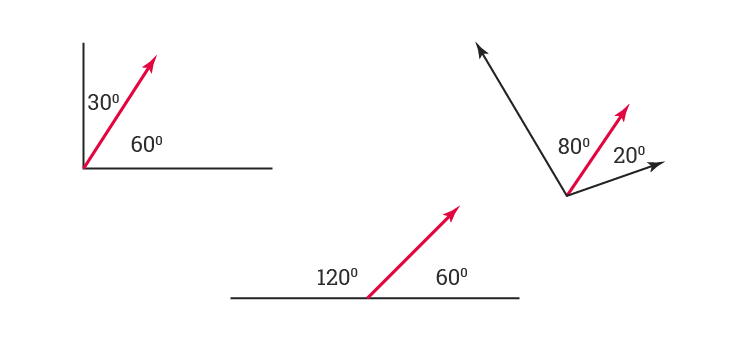### Linear Pair

Pair of adjacent angles whose measures add up to form a straight angle is known as a linear pair. The angles in a linear pair are supplementary.

Consider the following figure in which a ray $\overrightarrow{OP}$ stand on the line segment $\overline{AB}$  as shown: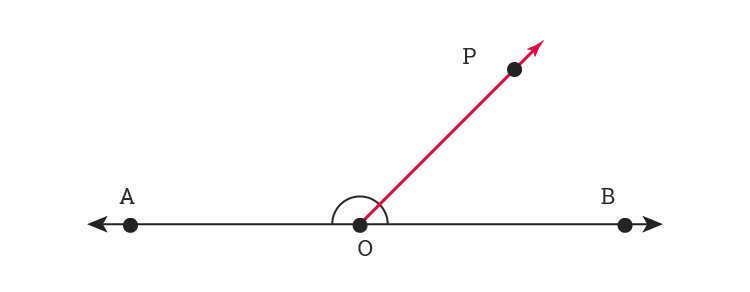The angles ∠POB and ∠POA are formed at O. ∠POB and ∠POA are adjacent angles and they are supplementary i.e. ∠POB + ∠POA = ∠AOB = 180°

∠POB and ∠POA are adjacent to each other and when the sum of adjacent angles is 180° then such angles form a linear pair of angles.

### Vertically Opposite Angles

When a pair of lines intersect, as shown in the fig. below, four angles are formed. ∠AOD and ∠COB are vertically opposite to each other and ∠AOC and ∠BOD are vertically opposite to each other. These angles are also known as vertical angles or opposite angles.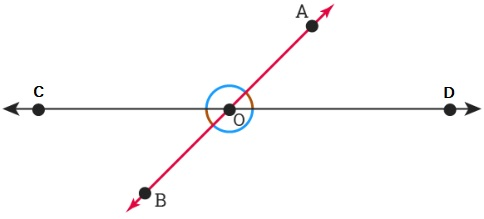Thus, when two lines intersect, two pair of vertically opposite angles are formed i.e. ∠AOD, ∠COB and ∠AOC, ∠BOD.

According to vertical angle theorem, in a pair of intersecting lines, the vertically opposite angles are equal.

Example 1: Find the value of x.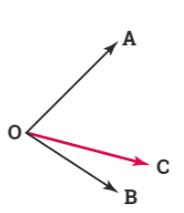If m∠AOB = 110° , m∠AOC = x and m∠ COB = 70°

Solution:

From figure:

m∠AOB = 110°

m∠AOC = x

m∠ COB = 70°

Now, from figure: ∠AOB = ∠AOC + ∠COB

m∠AOB = m∠AOC + m∠COB

110° = x + 70°

x = 110° – 70°

x = 30°

Example 2:

In the given figure, is ∠1 adjacent to ∠2. Give justification.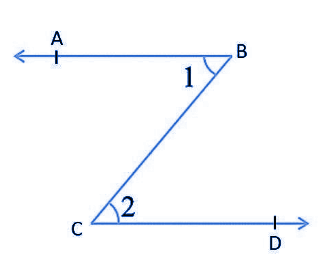Solution:

In the given figure, ∠1 does not share the vertex of ∠2.

As it does not obey the important property of adjacent angles,

∠1 is not adjacent to ∠2.